UIHistories Project: A History of the University of Illinois by Kalev Leetaru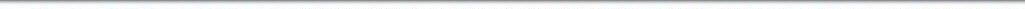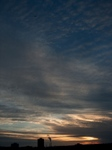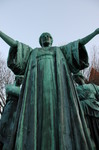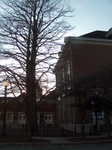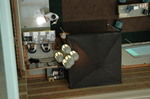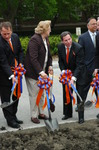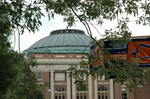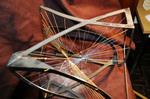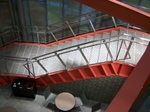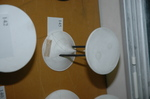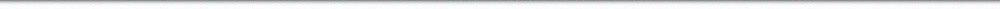N A V I G A T I O N D I G I T A L L I B R A R Y

## Repository: UIHistories Project: Booklet - Information on UI Chicago Professional Schools (1937) [PAGE 6]

Caption: Booklet - Information on UI Chicago Professional Schools (1937)
This is a reduced-resolution page image for fast online browsing.

< Previous Page [Displaying Page 6 of 17] Next Page >
[VIEW ALL PAGE THUMBNAILS]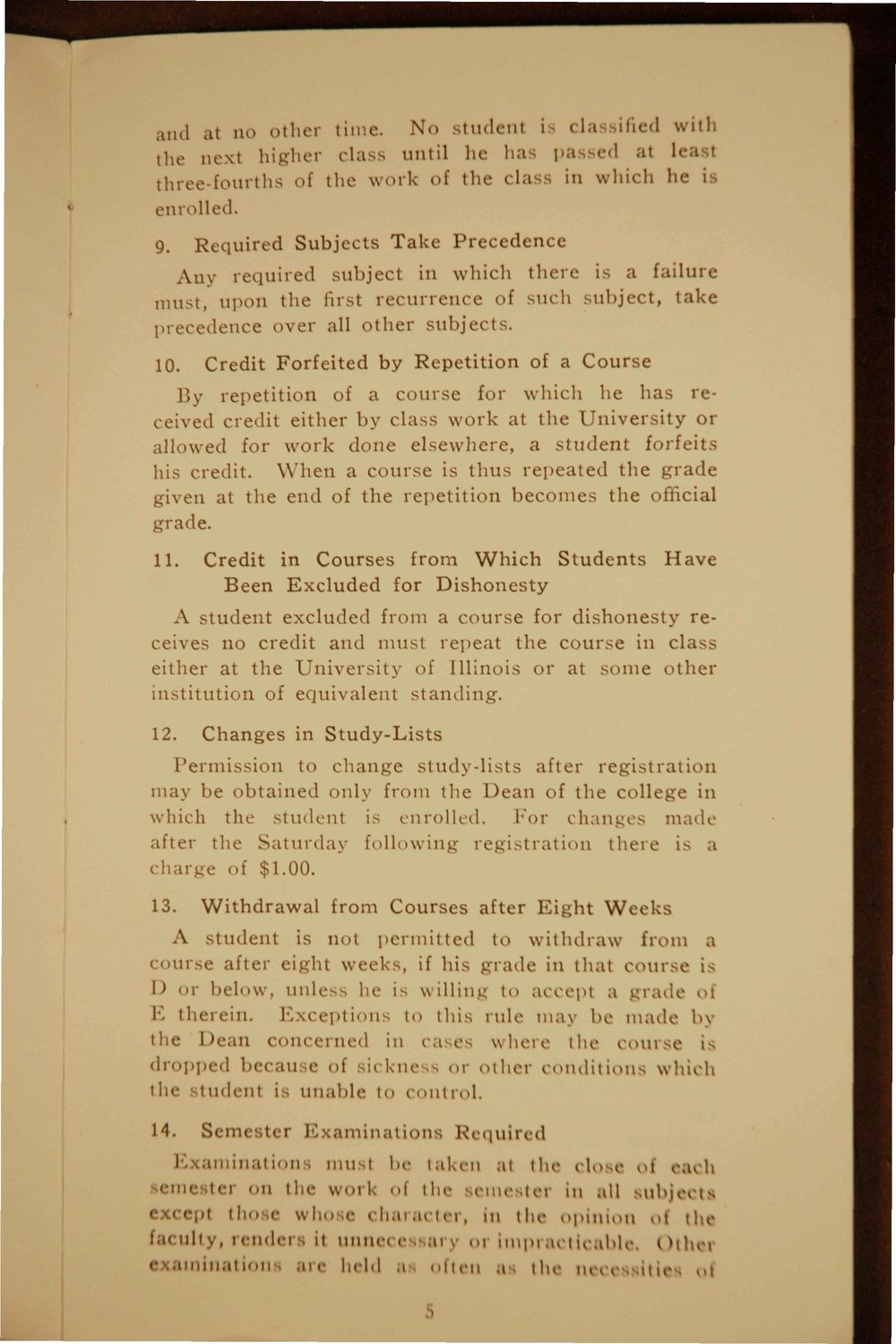### EXTRACTED TEXT FROM PAGE:

md at no other time.

No student i cla ified with

the next higher class until he has passed at lea three-fourths of the w o r k of tlie class in which he i enrolled. 9. Required Subjects T a k e Precedence A n y r e q u i r e d subject in which t h e r e is a failure must, upon the first r e c u r r e n c e of such subject, t a k e precedence over all o t h e r s u b j e c t s . 10. Credit F o r f e i t e d b y R e p e t i t i o n of a C o u r s e

By r e p e t i t i o n of a c o u r s e for which he h a s received credit either by class w o r k at t h e U n i v e r s i t y or allowed for w o r k d o n e e l s e w h e r e , a s t u d e n t forfeit his credit. W h e n a c o u r s e is t h u s r e p e a t e d t h e g r a d e given at t h e end of t h e r e p e t i t i o n b e c o m e s t h e official grade. 11. Credit in C o u r s e s from W h i c h S t u d e n t s B e e n E x c l u d e d for D i s h o n e s t y Have

A s t u d e n t excluded from a c o u r s e for d i s h o n e s t y receives no credit and must repeat t h e c o u r s e in class either at t h e U n i v e r s i t y of Illinois or at s o m e o t h e r i n s t i t u t i o n of e q u i v a l e n t s t a n d i n g . 12. C h a n g e s in S t u d y - L i s t s

Permission to c h a n g e s t u d y - l i s t s after r e g i s t r a t i o n may be o b t a i n e d only from t h e D e a n of t h e college in which the student is enrolled. For c h a n g e s m a d e after the S a t u r d a y following r e g i s t r a t i o n t h e r e is i h irge of \$1.00. 13. W i t h d r a w a l from C o u r s e s after E i g h t W e e k s

A s t u d e n t is not p e r m i t t e d to w i t h d r a w from a ourse after eight w e e k s , if his g r a d e in that c o u r s e is I) or below, unless he is willing to accept a g r a d e of E therein. E x c e p t i o n s to this rule may be m a d e b \

tlie Dean concerned in cases where the

nirse is

dropped becati e of sickne ,s or o t h e r c o n d i t i o n s which the student is u n a b l e to COlltl "I. 14. Semest< r . l a m i n a t i o n s Requiid

Examinations must be taken at the close ol each emester on the work < i the semester in all subjects > • ccepl those who e character, in the opinion ol th

faculty, l e n d e r s it unnec< ary 01 impracti< ihlc. I >thei

examination

are held a often a . the m 11 ,n . uj